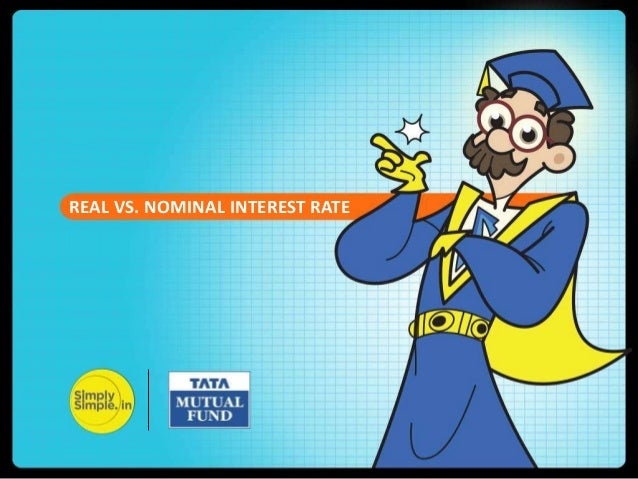Successfully reported this slideshow.Upcoming SlideShare
×

# Understanding real vs. nominal interest

4,286 views

Published on

Many of us receive calls from telemarketers selling lifetime free credit cards.

After understanding the product features, we straight away jump to the most important question.

Are there any hidden charges or is it really free?

Likewise, when it comes to interest rate, we should look at the hidden element – ‘Inflation’.

Inflation is a silent killer who eats majority portion of your income.

This lesson by Prof. Simply Simple attempts to explain the relationship between Nominal Interest Rate, Real Interest Rate and Inflation.

Look forward to your valuable feedback at professor@tataamc.com

Published in: Economy & Finance, Business
• Full Name
Comment goes here.

Are you sure you want to Yes No• Sex in your area is here: ❤❤❤ http://bit.ly/2Qu6Caa ❤❤❤

Are you sure you want to  Yes  No
• Dating direct: ❤❤❤ http://bit.ly/2Qu6Caa ❤❤❤

Are you sure you want to  Yes  No

### Understanding real vs. nominal interest

1. 1. REAL VS. NOMINAL INTEREST RATE
2. 2. The term “interest rate” is one of the most commonly used phrases in day to day finance. Today we will cover - Real vs. Nominal Interest Rates. REAL INTEREST RATE VS. NOMINAL INTEREST RATE
3. 3. Let us see the formula of the Current Account Balance (CAB) CAB = X - M + NI + NCT X = Exports of goods and services M = Imports of goods and services NI = Net income abroad [Salaries paid or received, credit / debit of income from FII & FDI etc. ] NCT = Net current transfers [Workers' Remittances (unilateral), Donations, Aids & Grants, Official, Assistance and Pensions etc] CURRENT ACCOUNT DEFICIT The difference between nominal and real interest rate is the key economic factor – ‘Inflation’. REAL INTEREST RATE VS. NOMINAL INTEREST RATE
4. 4. Inflation is a rise in the general price level. For instance, a 6% inflation rate means that an average basket of goods you purchased this year is 6% more expensive as compared to last year. REAL INTEREST RATE VS. NOMINAL INTEREST RATE
5. 5. REAL INTEREST RATE VS. NOMINAL INTEREST RATE Real Interest Rate is one where the effects of inflation have been factored in. Nominal Interest Rate is one where the effects of inflation have not been accounted for.
6. 6. Let us consider an example to illustrate the difference : REAL INTEREST RATE VS. NOMINAL INTEREST RATE
7. 7. REAL INTEREST RATE VS. NOMINAL INTEREST RATE Suppose your fixed deposit of Rs. 100 earns 10% interest at the end of the year. This means the Rs. 100 at beginning of the year will become Rs. 110 at the end of the year. This 10% is the nominal interest rate, as we have not accounted for inflation.
8. 8. Let us see the formula of the Current Account Balance (CAB) CAB = X - M + NI + NCT X = Exports of goods and services M = Imports of goods and services NI = Net income abroad [Salaries paid or received, credit / debit of income from FII & FDI etc. ] NCT = Net current transfers [Workers' Remittances (unilateral), Donations, Aids & Grants, Official, Assistance and Pensions etc] CURRENT ACCOUNT DEFICIT Whenever people speak of the interest rate they're talking about the nominal interest rate, unless they state otherwise. REAL INTEREST RATE VS. NOMINAL INTEREST RATE
9. 9. Assume that inflation rate is 6% for the year and your fixed deposit earns 10% nominal interest rate. After factoring in inflation, your deposit will earn a real interest rate of 4%. REAL INTEREST RATE VS. NOMINAL INTEREST RATE
10. 10. COMPARING REAL & NOMINAL INTEREST RATE REAL INTEREST RATE VS. NOMINAL INTEREST RATE Nominal Interest Rate = 10% Inflation = 6% Real Interest Rate = 4%
11. 11. Let us see the formula of the Current Account Balance (CAB) CAB = X - M + NI + NCT X = Exports of goods and services M = Imports of goods and services NI = Net income abroad [Salaries paid or received, credit / debit of income from FII & FDI etc. ] NCT = Net current transfers [Workers' Remittances (unilateral), Donations, Aids & Grants, Official, Assistance and Pensions etc] CURRENT ACCOUNT DEFICIT The relationship between nominal interest rate, inflation, and real interest rate is described by the Fisher Equation: REAL INTEREST RATE VS. NOMINAL INTEREST RATE Real Interest Rate = Nominal Interest Rate – Inflation
12. 12. If inflation is positive, then the real interest rate is lower than the nominal interest rate. If we have deflation and the inflation rate is negative, then the real interest rate will be larger. REAL INTEREST RATE VS. NOMINAL INTEREST RATE
13. 13. Let us see the formula of the Current Account Balance (CAB) CAB = X - M + NI + NCT X = Exports of goods and services M = Imports of goods and services NI = Net income abroad [Salaries paid or received, credit / debit of income from FII & FDI etc. ] NCT = Net current transfers [Workers' Remittances (unilateral), Donations, Aids & Grants, Official, Assistance and Pensions etc] CURRENT ACCOUNT DEFICIT Hope you have understood the difference between Real and Nominal Interest Rate. REAL INTEREST RATE VS. NOMINAL INTEREST RATE